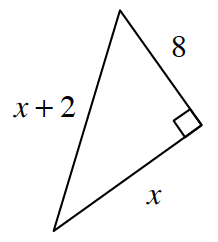### Home > CCG > Chapter 12 > Lesson 12.1.1 > Problem12-10

12-10.Examine the diagram at right.

1. Write an equation using the geometric relationships in the diagram. Then solve your equation for $x$.

Use the Pythagorean Theorem.

$\begin{array}{l} x^2+8^2=(x+2)^2 \\ x^2+64=x^2+4x+4 \\ 60=4x \\ x=15 \end{array}$

2. Find the measures of the acute angles of the triangle. What tool(s) did you use?

What trig ratio can you use to find one of the angles?
Once you have two angles, what relationship can you use to find the last angle?

$28.1°$$61.9°$